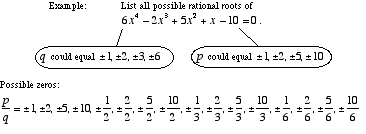index: click on a letter A B C D E F G H I J K L M N O P Q R S T U V W X Y Z A to Z index index: subject areas numbers & symbols sets, logic, proofs geometry algebra trigonometry advanced algebra & pre-calculus calculus advanced topics probability & statistics real world applications multimedia entrieswww.mathwords.com about mathwords website feedback

 Rational Root Theorem Rational Zero Theorem A theorem that provides a complete list of possible rational roots of the polynomial equation anxn + an–1xn–1 + ··· + a2x2 + a1x + a0 = 0 where all coefficients are integers. This list consists of all possible numbers of the form c/d, where c and d are integers. c must divide evenly into the constant term a0. d must divide evenly into the leading coefficient an.See also Polynomial facts